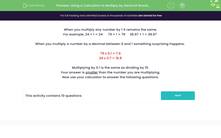# Use a Calculator to Multiply by Decimal Numbers Between 0 and 1

In this worksheet, students will use a calculator to multiply by decimal numbers between 0 and 1.Key stage:  KS 3

Curriculum topic:   Number

Curriculum subtopic:   Use Calculators/Technology for Accuracy

Difficulty level:#### Worksheet Overview

When you multiply any number by 1 it remains the same.

For example, 24 × 1 = 24      79 × 1 = 79     38.97 × 1 = 38.97

When you multiply a number by a decimal between 0 and 1 something surprising happens.

79 x 0.1 = 7.9

24 x 0.7 = 16.8

Multiplying by 0.1 is the same as dividing by 10.

This is a useful thing to know because otherwise you might think you've got the wrong answer!

For this activity, we are going to use a calculator to answer the following questions.

### What is EdPlace?

We're your National Curriculum aligned online education content provider helping each child succeed in English, maths and science from year 1 to GCSE. With an EdPlace account you’ll be able to track and measure progress, helping each child achieve their best. We build confidence and attainment by personalising each child’s learning at a level that suits them.

Get started